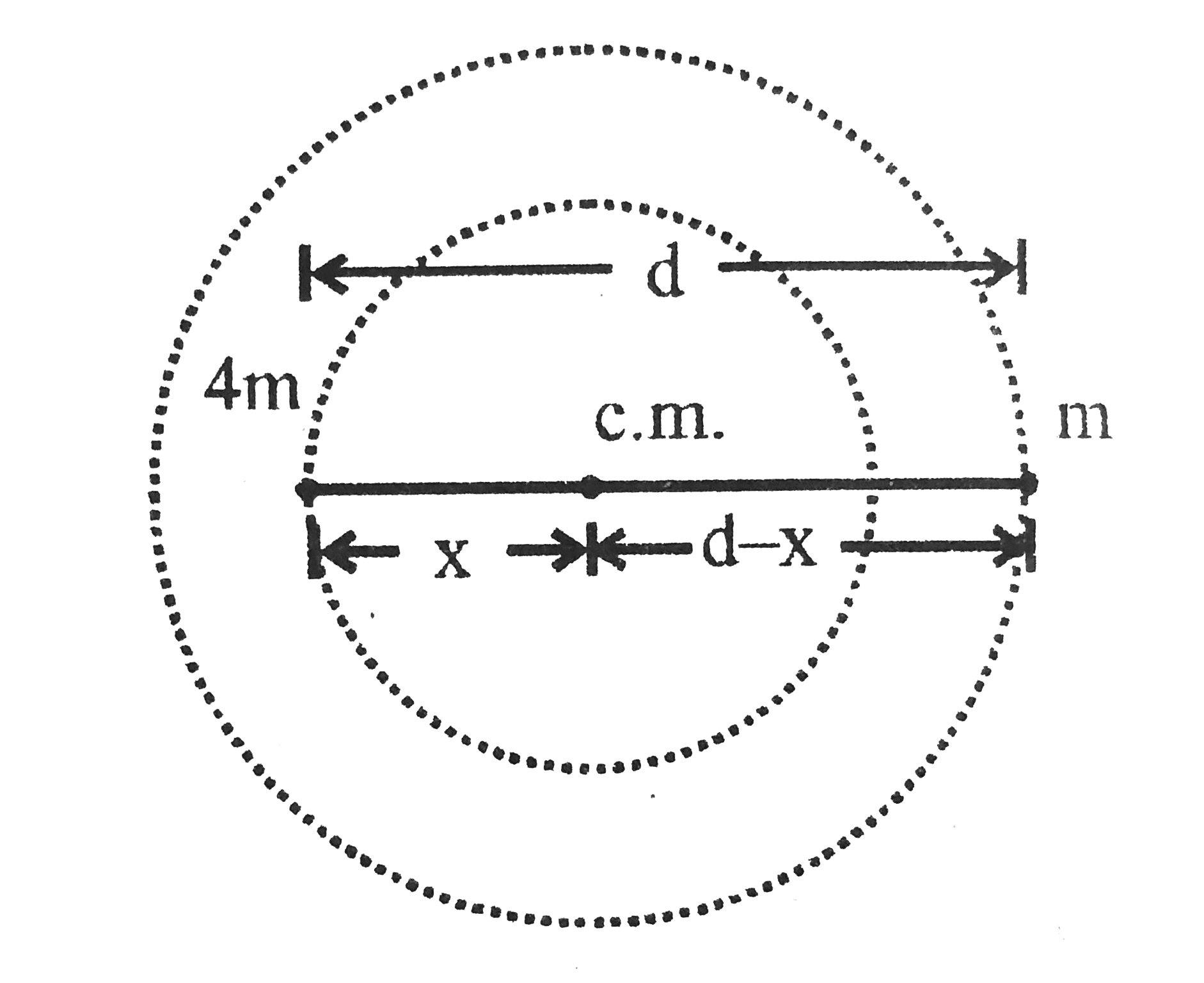# Two point masses of mass 4m and m respectively separated by d distance are revolving under mutual force of attraction. Ration of their kinetic e

25 views
in Physics
closed
Two point masses of mass 4m and m respectively separated by d distance are revolving under mutual force of attraction. Ration of their kinetic energies will be:
A. 1:4
B. 1:5
C. 1:1
D. 1:2

by (74.9k points)
selected

Correct Answer - a
They will revolue about this centre of mass position of centre of mass
0=4m(-x)+m(d-x)
x=d/5
They will same  omega
(K_(4m))/(K_(m))=(1/2I_(4m)omega^(2))/(1/2I_(m)omega^(2))
(K_(4m))/(K_(m))=(1/2(4m)(d//5)^(2))/(1/2(m)(4d//5)^(2))implies(K_(4m))/(K_(m))=1/4